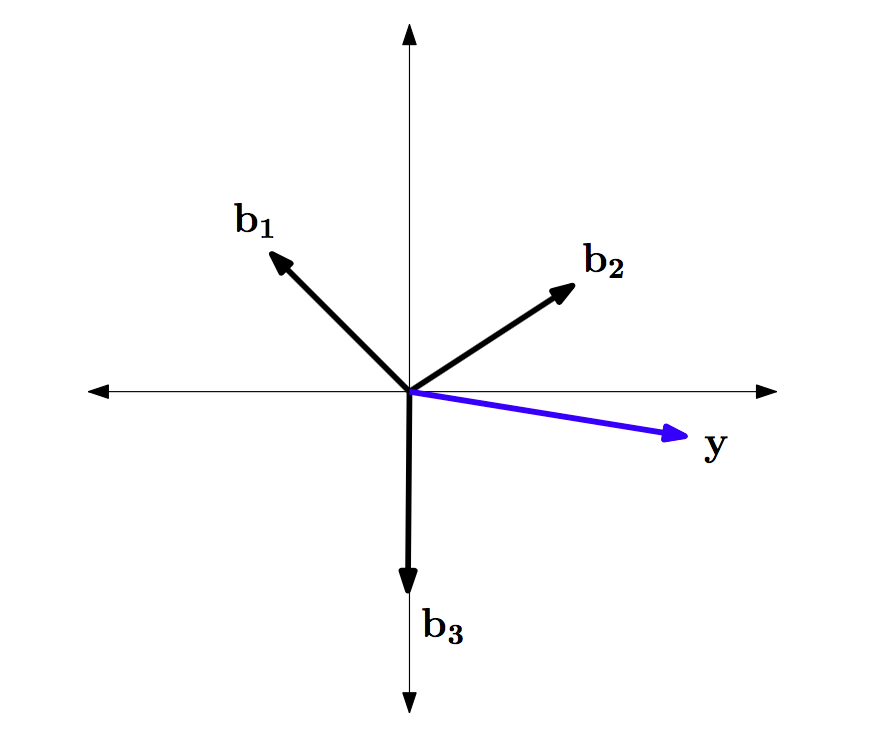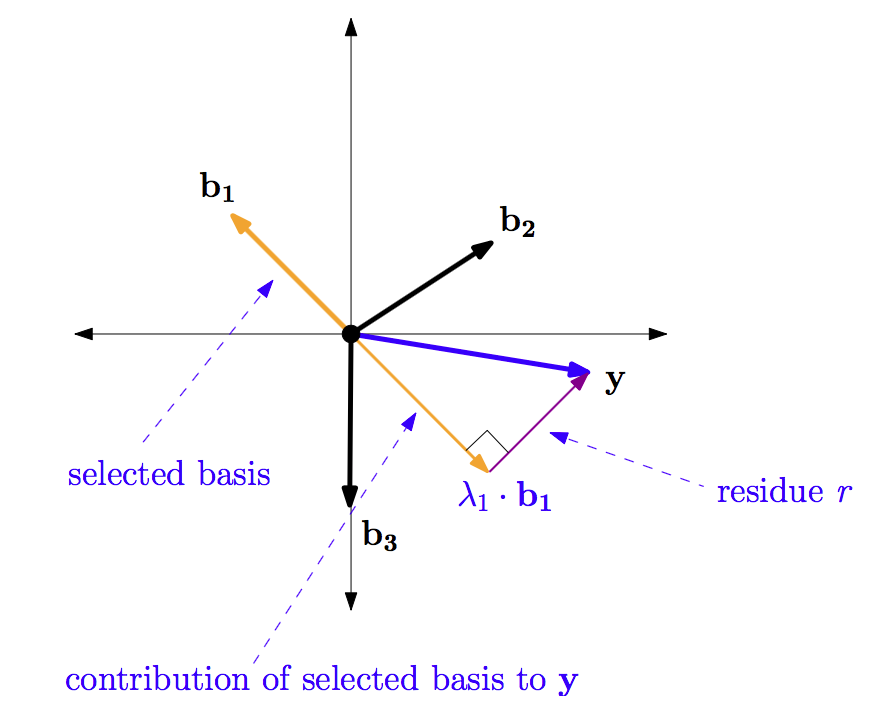10 篇文章 0 订阅

# 简介

y=Ax=[0.7070.7070.80.601][1.210]=[1.650.25]

# 基本概念

A=[0.7070.7070.80.601]=[b1b2b3]

b1=[0.7070.707],b2=[0.80.6],b1=

Ax=[0.7070.707]x1+[0.80.6]x2+x3=y
.

OMP算法和MP算法类似，都是从字典中找出哪一个原子对 y $y$值的贡献最大，接下来是哪个原子的贡献值大，以此类推. 我们现在知道这个过程需要N$N$次迭代， N $N$是字典中原子的个数. 在该例子中，N$N$等于3.

# 计算原子的贡献值

b1=[0.7070.707],b2=[0.80.6],b1=

y=[1.650.25]

<b1,y>=[0.7070.707]T[1.650.25]=0.7071.65+0.7070.25=1.34

<b2,y>=1.17

<b3,y>=0.25 <script type="math/tex" id="MathJax-Element-41"> = 0.25</script>

w=ATy=0.7070.800.7070.61[1.650.25]=1.341.170.25# 计算残差

r=yλ1b1=[1.650.25](1.34)[0.7070.707]=[0.70.7]# 重复迭代

Anew=[b1]=[0.7070.707]

w=[b2b3]Tr=[0.980.7]

Anew=[b1b2]=[0.7070.7070.80.6]

minAnewλy2

min[b1b2]=[0.7070.7070.80.6][λ1λ2][1.650.25]2

λ=A+newy

（注：可参看我的博文《Moore-Penrose广义逆矩阵》和《矛盾方程的最小二乘解法》）

λ=A+newy=[0.60620.71430.80820.7143][1.650.25]=[1.21] $\mathbf{\lambda} = \mathrm{A}_{new}^+ \cdot y = \begin{bmatrix}-0.6062 & 0.8082 \\ 0.7143 & 0.7143\end{bmatrix} \cdot \begin{bmatrix}1.65 \\ -0.25\end{bmatrix} = \begin{bmatrix}-1.2 \\ 1\end{bmatrix}$

xrec=1.210 $x_{rec} = \begin{bmatrix}-1.2 \\ 1 \\ 0\end{bmatrix}$

r=yAnewλ=[1.650.25][0.7070.7070.80.6][1.21]= $r = y - \mathrm{A}_{new} \cdot \mathbf{\lambda} = \begin{bmatrix}1.65 \\ -0.25\end{bmatrix} - \begin{bmatrix}-0.707 & 0.8 \\ 0.707 & 0.6\end{bmatrix} \cdot \begin{bmatrix}—1.2 \\ 1\end{bmatrix} = \begin{bmatrix}0 \\ 0\end{bmatrix}$

# 其他例子

1. 我们有4个基（原子）： b1=0.80.20.2b2=0.30.41b3=10.30.1b4=0.40.40.8 $b_1 = \begin{bmatrix}-0.8 \\ -0.2 \\ 0.2\end{bmatrix} \qquad b_2 = \begin{bmatrix}0.3 \\ 0.4 \\ 1\end{bmatrix} \qquad b_3 = \begin{bmatrix}1 \\ -0.3 \\ -0.1\end{bmatrix} \qquad b_4 = \begin{bmatrix}0.4 \\ -0.4 \\ 0.8\end{bmatrix} \qquad$

2. 由于基向量的长度不是1，所以我们首先进行标准化.

b1^=b1/b1=0.80.20.2/(0.8)2+(0.4)2+(0.2)2=0.94280.23570.2357

b2^=b2/b2=0.26800.35780.8940

b3^=b3/b3=0.95350.28600.0953

b2^=b2/b2=0.40820.40820.8165

3. 标准化的基向量对 y $y$的贡献w$w$

w=A^Ty=0.94280.23570.23570.26800.35780.98400.95350.28600.09530.40820.40820.8165T2.70.14.5=1.50854.78522.11674.7357

4. 第二个基向量 b2 $b_2$贡献值最大，所以将 b2 $b_2$加入到 Anew $\mathrm{A}_{new}$

Anew=b2=0.30.41 $\mathrm{A}_{new} = b_2 = \begin{bmatrix}0.3 \\ 0.4 \\ 1\end{bmatrix}$

5. 利用最小二乘法计算 xrec $x_{rec}$

Lp=A+newy=[4.28] $L_p = \mathrm{A}_{new}^+ \cdot y = [4.28]$

因为 Lp $L_p$对应着第二个基向量，所以 xrec=04.2800 $x_{rec} = \begin{bmatrix}0 \\ 4.28 \\ 0 \\ 0\end{bmatrix}$

6. 接下来计算残差

r=yAnewLp=2.70.14.50.30.414.28=1.4161.6120.22

7. 接下来重复第3步，计算 b1^ $\hat{b_1}$ b1^ $\hat{b_1}$ b1^ $\hat{b_1}$ r $r$的贡献.

w=A^Tr=0.94280.23570.23570.95350.28600.09530.40820.40820.8165T1.4161.6120.22=0.90321.79021.4158

选择第二个贡献最大的基 b3 $b_3$，其贡献值为1.7902.

8. 将选择的 b3 $b_3$加入到 Anew $\mathrm{A}_{new}$

Anew=[b1b2]=0.30.4110.30.1 $\mathrm{A}_{new} = \begin{bmatrix}b_1 & b_2\end{bmatrix} = \begin{bmatrix}0.3 & 1\\ 0.4 & -0.3\\ 1 & -0.1\end{bmatrix}$

9. 用最小二乘法计算 Lp=A+newy=[4.17021.7149] $L_p = \mathrm{A}_{new}^+ \cdot y = \begin{bmatrix}4.1702 \\ 1.7149\end{bmatrix}$

这个 Lp $L_p$对应着 b2 $b_2$ b3 $b_3$，因此 xrec=04.1721.71490 $x_{rec} = \begin{bmatrix}0 \\ 4.172 \\ 1.7149 \\ 0\end{bmatrix}$

10. 接着计算残差

r=yAnewLp=2.70.14.50.30.4110.30.1[4.1721.7149]=0.2661.05360.5012

11. 重复步骤2，进行最后一次迭代

12. 计算 b1 $b_1$ b4 $b_4$的贡献值 w=[b1^b2^]r=0.94280.23570.23570.40820.40820.8165T0.2661.05360.5012=[0.61720.7308] $w = \begin{bmatrix}\hat{b_1} & \hat{b_2}\end{bmatrix} \cdot r = \begin{bmatrix}-0.9428 & 0.4082 \\ -0.2357 & -0.4082\\ 0.2357 & -0.8165\end{bmatrix}^{\mathrm{T}} \cdot \begin{bmatrix}-0.266 \\ -1.0536 \\ 0.5012\end{bmatrix} = \begin{bmatrix}0.6172 \\ 0.7308\end{bmatrix}$

这里 b4 $b_4$提供了最大贡献值0.7308.

13. b4 $b_4$加入 Anew=0.30.4110.30.10.40.40.8 $\mathrm{A}_{new} = \begin{bmatrix}0.3 & 1 & 0.4 \\ 0.4 & -0.3 & -0.4 \\ 1 & -0.1 & 0.8\end{bmatrix}$

14. 利用最小二乘计算 Lp=A+newy=321 $L_p = \mathrm{A}_{new}^+ \cdot y = \begin{bmatrix}3 \\ 2 \\1\end{bmatrix}$，对应的 xrec=0312 $x_{rec} = \begin{bmatrix}0 \\ 3 \\ 1 \\ 2\end{bmatrix}$

15. 接着计算残差

r=yAnewLp=2.70.14.50.30.4110.30.10.40.40.8312=000

16. 我们的迭代到此为止，因为此时残差已经为0，重建的 x $x$xrec=0312$x_{rec} = \begin{bmatrix}0 \\ 3 \\1 \\2\end{bmatrix}$，和原来的信号相同.

# 需要注意的问题

1. OMP中最大贡献值的计算需要对基向量进行标准化处理，不是由原始基得到的.
2. 如果给定的基向量已经是单位向量，则不需要进行标准化.
3. 基向量对 y $y$值的贡献的计算是通过标准化以后的基向量和残差的点积进行计算的.
4. 在MP算法中，重建系数xrec$x_{rec}$的计算是通过基向量和残差的点积进行计算的，在OMP算法中， xrec $x_{rec}$的计算是通过最小二乘法得到 Anew $\mathrm{A}_{new}$相对于 y $y$的系数得到的，即Lp$L_p$的计算. Lp $L_p$向量中的值直接对应于 xrec $x_{rec}$的相应位置. Anew $\mathrm{A}_{new}$通过每次对基向量的选择得到. 这个过程是需要时间的，因此OMP比MP慢. （不是应该OMP收敛的快吗？）
5. 残差 r $r$的计算通过原始的y$y$值和 Anew $\mathrm{A}_{new}$ Lp $L_p$得到.
6. 迭代的次数最多等于 A $\mathrm{A}$矩阵的行数M，或者如果给定了稀疏度 K $K$，则迭代K$K$次. 如果 K<M $K < M$，则已知的 K $K$可以加快计算结束，如果K$K$未知，则迭代 M $M$次.

# 说明总结

OMP算法 步骤描述：

1. 找出残差 r $r$和字典矩阵的列Ai$\mathrm{A}_i$积中最大值所对应的脚标 λ $\lambda$，即 λt=argmaxi=1,,N|<rt1,Ai>| $\lambda_t = \arg \max_{i=1,\cdots, N}\left|\right|$.
2. 更新索引集 Λt=Λt1{λt} $\Lambda_t = \Lambda_{t-1} \cup \{\lambda_t\}$，记录找到的字典矩阵中的重建原子集合 At=[At1,Aλt] $A_t = [A_{t-1}, A_{\lambda_t}]$.
3. 由最小二乘得到 x^t=argmin|yAtx^| $\hat{x}_t = \arg \min \left|| y - A_t \hat{x} |\right|$.
4. 更新残差 rt=yAtx^t $r_t = y - A_t \hat{x}_t$ t=t+1 $t=t+1$.
5. 判断是否满足 t>K $t > K$，若满足，则迭代停止；若不满足，则继续执行步骤1.
03-04
05-133万+04-1715万+
04-199万+
06-09611
09-11
09-19
02-201万+
07-11
04-171万+
05-231518
09-153589
04-05点击重新获取扫码支付余额充值